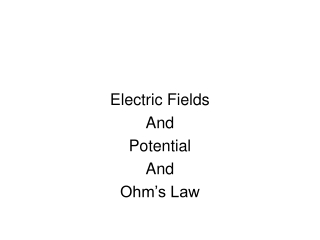DownloadDownload PresentationElectric Fields And Potential And Ohm’s Law

# Electric Fields And Potential And Ohm’s Law

Download Presentation## Electric Fields And Potential And Ohm’s Law

- - - - - - - - - - - - - - - - - - - - - - - - - - - E N D - - - - - - - - - - - - - - - - - - - - - - - - - - -
##### Presentation Transcript

1. Electric Fields And Potential And Ohm’s Law

2. Just as the space around Earth and every other mass is filled with a gravitational field, the space around every electric charge is filled with an electric field.

3. Electric fieldThe area around a charged object. This field exerts a force on any charged object in its vicinity. The closer the charged object is brought to the charged object creating the field, the greater the force exerted on it.

4. VectorIts magnitude (strength) can be measured by its effect on charges located in the field.

5. Electric field linesThe electric field can be represented with electric field lines. The closer the lines are, the stronger the field is.

6. electric field lines point in the direction which a positive test charge would accelerate if placed upon the line.

7. Field lines are always directed from the positive charge and toward a negative charge.

8. Electric Potential Energy:The energy a charge possesses by virtue of its location

9. Electric Potentialwork done on a charge; or the electric potential is the potential energy per unit charge (symbol is V; SI unit is volt)

10. Electric Potential = Electrical potential energy / charge

11. Potential = work / test chargeorV = W / qo

12. Remember, the term “work” can be replaced with the term “energy” because to store energy in, or to give energy to, an object, work must be done.

13. 1 volt = 1 Joule / coulomb

14. Capacitora device that stores charge in the electric field between its plates. Each plate carries the same amount of charge, one plate being negative and the other being positive. A potential difference exists between the two plates.

15. Capacitors are found in almost all electronic circuits

16. Ohm’s LawGeorg Simon Ohm discovered that the current in a circuit is directly proportional to the voltage impressed across the circuit and is inversely proportional to the resistance of the circuit

17. Current = voltage / resistanceI = V / RCurrent (I) – amperesPotential Difference (V) – voltsResistance (R) - ohms